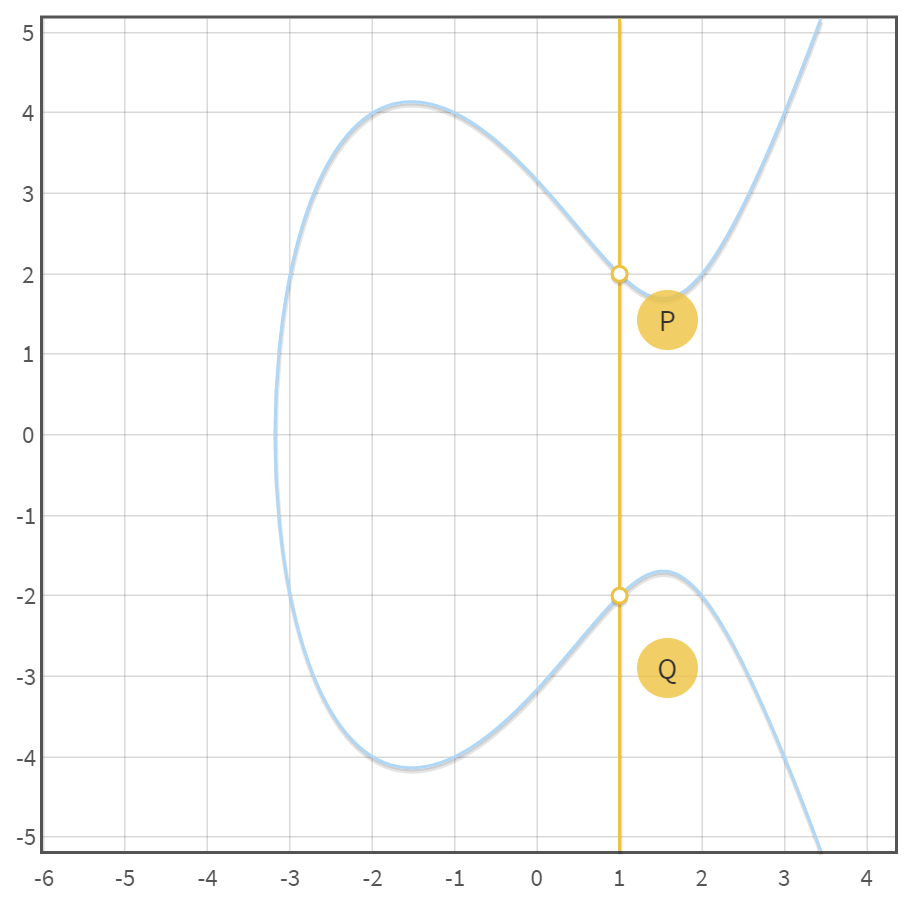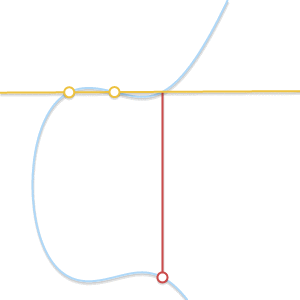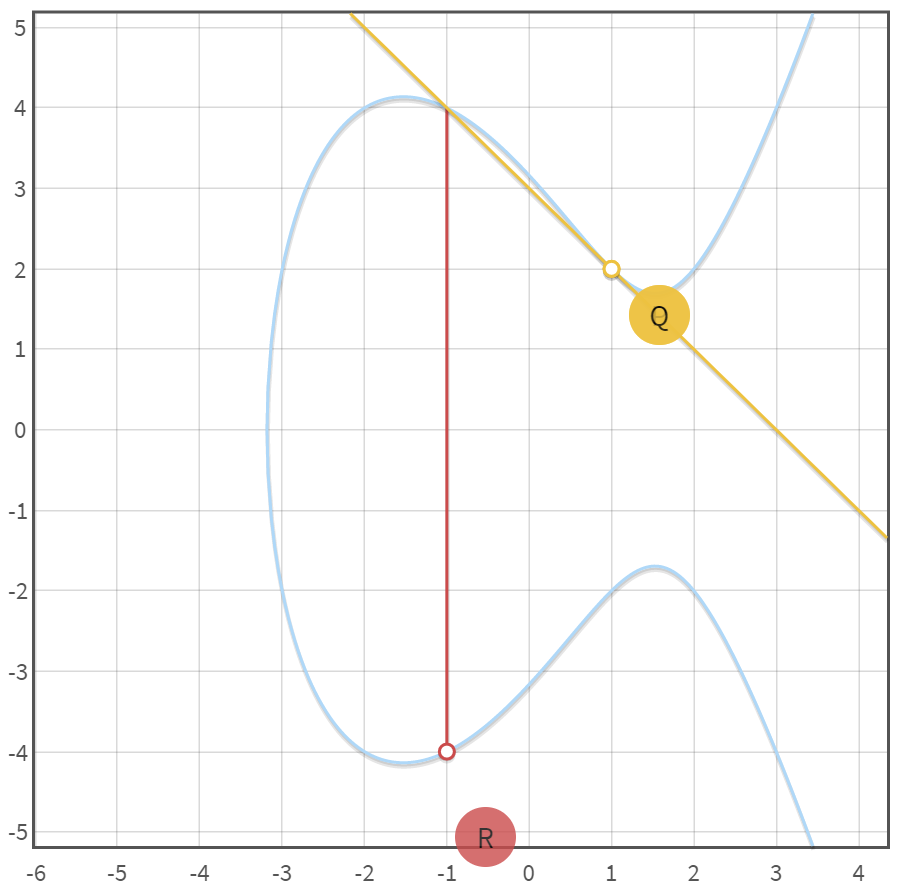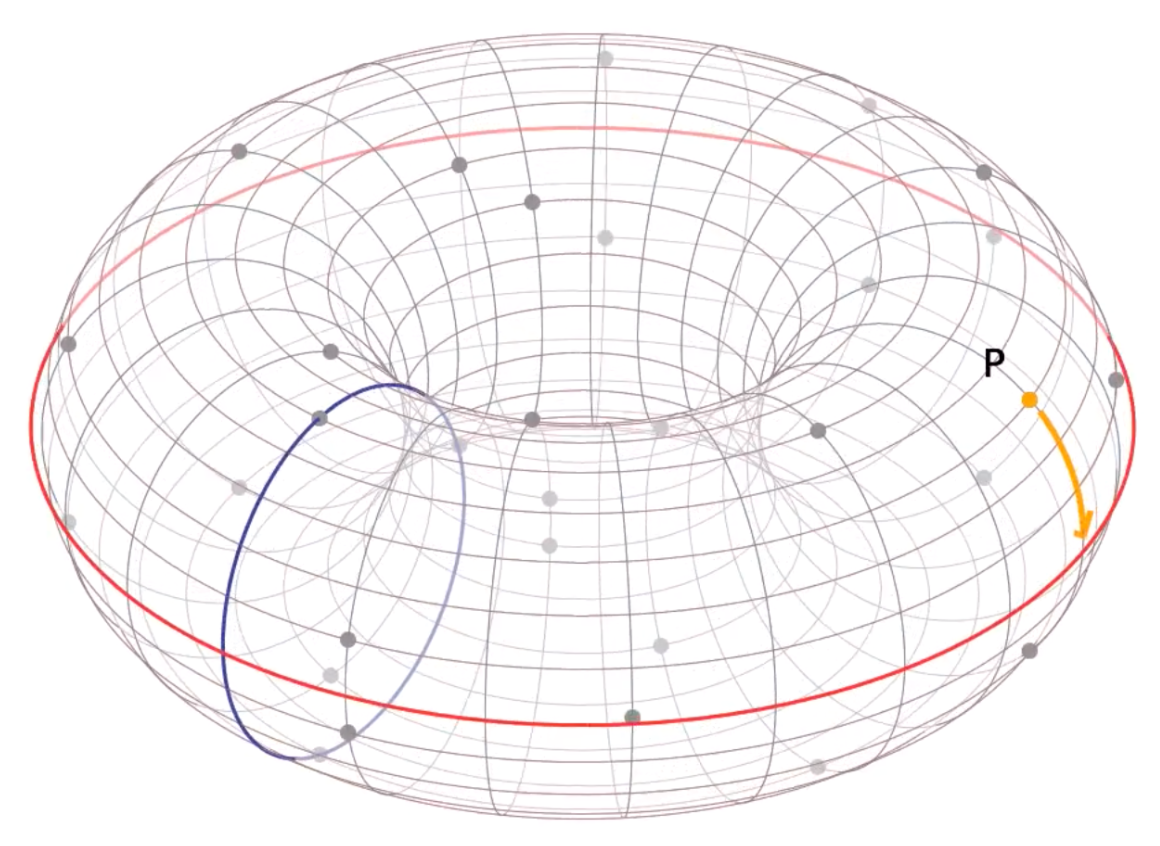https://www.interchains.cc/23536.html

• samczsun
• ECDSA

1. 零知识证明 – 椭圆曲线基础
2. Elliptic curves over real numbers and the group law
3. Elliptic curves over finite fields and the discrete logarithm problem
4. Key pair generation and two ECC algorithms: ECDH and ECDSA
5. Algorithms for breaking ECC security, and a comparison with RSA

## ECC, ECDSA

ECC是Elliptic Curve Cryptography的缩写，ECDSA是基于ECC的一种签名算法。

## 实数域上的ECC

\$\$ y^2=x^3+ax+b 其中：4a^3+27b^2 neq 0 \$\$

\$\$ left{ (x, y) in mathbb{R}^2 | y^2 = x^3 + ax + b, 4 a^3 + 27 b^2 ne 0 right} cup left{ 0 right} \$\$

### 加法

\$\$ P + (Q + R) = Q + (P + R) = R + (P + Q) = cdots = 0 \$\$

#### case 1: P点和Q点关于X轴对称

\$\$ P+(-P)=0 \$\$#### case2: P点和Q点是同一个点

\$\$ P+P=-R \$\$### 乘法

\$\$ nP = underbrace{P + P + cdots + P}_{n text{times}} \$\$\$\$ 151 = 1 cdot 2^7 + 0 cdot 2^6 + 0 cdot 2^5 + 1 cdot 2^4 + 0 cdot 2^3 + 1 cdot 2^2 + 1 cdot 2^1 + 1 cdot 2^0 = 2^7 + 2^4 + 2^2 + 2^1 + 2^0 \$\$

\$\$ 151 cdot P = 2^7 P + 2^4 P + 2^2 P + 2^1 P + 2^0 P 2^0 P = P 2^1 P = P+P 2^2 P = 2^1P + 2^1P 2^3 P = 2^2P + 2^2P 2^4 P = 2^3P + 2^3P 2^5 P = 2^4P + 2^4P 2^6 P = 2^5P + 2^5P 2^7 P = 2^6P + 2^6P \$\$

## 有限域上的ECC

### 有限域上的ECC定义

\$\$ begin{array}{rcl} left{(x, y) in (mathbb{F}_p)^2 right. & left. | right. & left. y^2 equiv x^3 + ax + b pmod{p}, right. & & left. 4a^3 + 27b^2 notequiv 0 pmod{p}right} cup left{0right} end{array} \$\$

### 有限域的加法：### 有限域的乘法

\$\$ n P = underbrace{P + P + cdots + P}_{n text{times}} \$\$

### 局部有限域的点的数量

subgroup order对以太坊eth是一个很重要的概念，因为ECDSA签名就是基于一个subgroup order， 而不是一个完整的有限域的点。所以我们需要知道ECC对应的局部有限域的独立的点的数量。

### 选取最优基点

\$\$ Ntimes P=0 \$\$

\$\$ ntimes htimes P=0 \$\$

## ECDSA

### 椭圆曲线的域名参数

``指明有限域大小的素数: P=0xFFFFFFFF FFFFFFFF FFFFFFFF FFFFFFFF FFFFFFFF FFFFFFFF FFFFFFFE FFFFFC2F 椭圆曲线方程的系数: a = 0, b=7 产生我们子群的基点 base point: G(x,y) G[x] = 0x79BE667E F9DCBBAC 55A06295 CE870B07 029BFCDB 2DCE28D9 59F2815B 16F81798 G[y] = 0x483ADA77 26A3C465 5DA4FBFC 0E1108A8 FD17B448 A6855419 9C47D08F FB10D4B8 子群的order:  n=0xFFFFFFFF FFFFFFFF FFFFFFFF FFFFFFFE BAAEDCE6 AF48A03B BFD25E8C D0364141 子群的辅助因子 h=1``

### 私钥

\$\$ Pri < n Pub_x = Pritimes G_x Pub_y=Pritimes G_y \$\$

### 公钥

\$\$ PUB=Pub_xcdot pub_y \$\$

### ECDSA交易签名函数

ECDSA是一种对交易信息的哈希值进行签名的函数，当Alice对交易签名后得到(r,s), 任何人都可以利用(r,s) 以及相应的交易信息的哈希值运算得到Alice的公钥，从而验证该交易是否是Alice发出的。

``alice 构造一笔交易，交易信息如下： [nonce,gasPrice,gasLimit,to,value,data,chainId,0,0] => [0,0x09184e72a000,0x30000,0xb0920c523d582040f2bcb1bd7fb1c7c1ecebdb34, 0, "",1,0,0] RLP编码后得到的数据为： 其为list，按照list编码要求编码 nonce: 0 => 0x80 => len = 0x01 gasPrice: 0x09184e72a000 => 0x8609184e72a000 => len = 0x07 gasLimit: 0x30000 => 0x83030000 => len=0x04 to: 0xb0920c523d582040f2bcb1bd7fb1c7c1ecebdb34 => 0x94b0920c523d582040f2bcb1bd7fb1c7c1ecebdb34 => len = 0x15 value: 0 => 0x80 => len = 0x01 data: "" => 0x80 => len = 0x01 chainId,0,0 => 0x018080 => len = 0x03 最后整合为list，编码为: 0xe6808609184e72a0008303000094b0920c523d582040f2bcb1bd7fb1c7c1ecebdb348080018080 需要签名的交易哈希为： keccak256(RLP([nonce...]))=901237a23fc304ddebc9d4f103a19bacc9fd7294bb1b7206a65cafa420804afa``

\$\$ P=kcdot G \$\$

\$\$ r=P_x % n \$\$

\$\$ s=k^{-1}cdot(rtimes d_A+z) % n \$\$

### ECDSA交易验证

\$\$ H_A=r^{-1}cdot (sR-zG) H_A’=r^{-1} cdot (sR’-zG) \$\$

\$\$ H_A = r^{-1} cdot (sR-zG) H_A = r^{-1} cdot (scdot kcdot G -zG) H_A = r^{-1} cdot (rtimes d_A cdot G + zG – zG ) H_A = r^{-1} cdot (r times H_A) H_A = H_A \$\$

## ECDSA的攻击利用

\$\$ s_1=k^{-1}(rcdot d_A+z_1) s_2 = k^{-1}(rcdot d_A+z_2) s_1-s_2=k^{-1}(z_1-z_2) k=(z_1-z_2)(s_1-s_2)^{-1} d_A=(s_1k-z_1)r^{-1} \$\$

``message? ok keccak256("ok")=14502d3ab34ae28d404da8f6ec0501c6f295f66caa41e122cfa9b1291bc0f9e8 r=0xeac250f3dccb2eef0e7c4112807a61815fc61f4d241d5076fcad1b50629e9671 s=0xc2a39d1efc8f7fca69a3099deda8c2fbd4304e6cfee6dba075e1b9a0fbfa999d message? hi keccak256("hi")=7624778dedc75f8b322b9fa1632a610d40b85e106c7d9bf0e743a9ce291b9c6f r=0xeac250f3dccb2eef0e7c4112807a61815fc61f4d241d5076fcad1b50629e9671 s=0xc070c31f27b4e4661b5a701d77bf5813e270b1ca28d7a56d366c30106f527b69``

``def modDivide(a,b,m):     a = a % m     inv = modInverse(b,m)     if (inv == -1):         print("Division not defined")     else:         return (inv*a) % m def modInverse(b, m):     g = math.gcd(b, m)     if (g != 1):         return -1     else:         return pow(b, m-2, m) def hash_mssage(msg: str) -> int:     # hash the message using keccak256, truncate if necessary     k = sha3.keccak_256()     k.update(msg.encode('utf8'))     d = k.digest()     n = int(binascii.hexlify(d),16)     olen = ecdsa.generator_secp256k1.order().bit_length() or 1     dlen = len(d)     n >>= max(0, dlen-olen)     return n if __name__ == "__main__":     # msg1 = input("msg1? ")     msg1 = "hi"     msg1_hashed = hash_mssage(msg1)     print(str(msg1_hashed))     # msg2 = input("msg2? ")     msg2 = "ok"     msg2_hashed = hash_mssage(msg2)     print(str(msg2_hashed))      m = ecdsa.generator_secp256k1.order()     # r1 = int(input("r1? "), 16)     r1 = int("0xeac250f3dccb2eef0e7c4112807a61815fc61f4d241d5076fcad1b50629e9671",16)     s1 = int("0xa8e72d180daf4d7f10778835719bb6de0abc003b94d71f28a99d673df4b06e17",16)     s2 = int("0xab1a0717e289e8e35ec021b5e78521c5fc7b9cde6ae6555be912f0ce81588c4b",16)     # s1 = int(input("s1? "), 16)     # s2 = int(input("s2? "), 16)      g = ecdsa.generator_secp256k1     k = modDivide((msg1_hashed - msg2_hashed), (s1 - s2),m)     d = modDivide(((s1 * k) - msg1_hashed), r1,m)     print("d_A: ", d)     test = input("test? ")     test_hashed = hash_mssage(test)     pub = ecdsa.Public_key(g, g * d)     priv = ecdsa.Private_key(pub, d)     sig = priv.sign(test_hashed, k)     print(f"solved r=0x{sig.r:032x}")     print(f"solved s=0x{sig.s:032x}")``